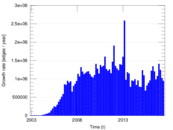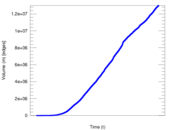# Wikipedia edits (cs)

This is the bipartite edit network of the Czech Wikipedia. It contains users and pages from the Czech Wikipedia, connected by edit events. Each edge represents an edit. The dataset includes the timestamp of each edit.

 Code `cs` Internal name `edit-cswiki` Name Wikipedia edits (cs) Data source http://dumps.wikimedia.org/ AvailabilityDataset is available for download Consistency checkDataset passed all tests Category Authorship network Dataset timestamp 2017-10-20 Node meaning User, article Edge meaning Edit Network formatBipartite, undirected Edge typeUnweighted, multiple edges Temporal dataEdges are annotated with timestamps

## Statistics

 Size n = 1,114,389 Left size n1 = 83,870 Right size n2 = 1,030,519 Volume m = 12,998,902 Unique edge count m̿ = 6,903,670 Wedge count s = 179,644,761,176 Claw count z = 9,920,289,916,460,502 Cross count x = 6.262 12 × 1020 Maximum degree dmax = 995,336 Maximum left degree d1max = 995,336 Maximum right degree d2max = 25,562 Average degree d = 23.329 2 Average left degree d1 = 154.989 Average right degree d2 = 12.613 9 Average edge multiplicity m̃ = 1.882 90 Size of LCC N = 1,097,632 Diameter δ = 16 50-Percentile effective diameter δ0.5 = 3.413 74 90-Percentile effective diameter δ0.9 = 3.959 64 Median distance δM = 4 Mean distance δm = 3.758 49 Balanced inequality ratio P = 0.158 713 Left balanced inequality ratio P1 = 0.043 286 4 Right balanced inequality ratio P2 = 0.214 355 Power law exponent γ = 1.904 84 Tail power law exponent γt = 2.901 00 Degree assortativity ρ = −0.068 730 8 Degree assortativity p-value pρ = 0.000 00 Spectral norm α = 5,147.27 Spectral separation |λ1[A] / λ2[A]| = 1.075 37 Controllability C = 963,998 Relative controllability Cr = 0.871 991

## Plots

### Degree distribution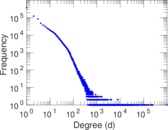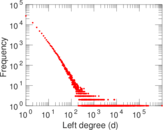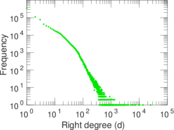### Cumulative degree distribution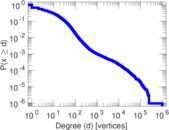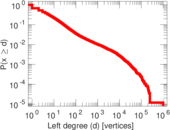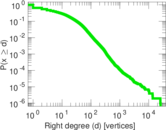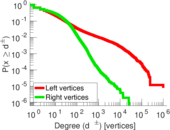### Spectral distribution of the adjacency matrix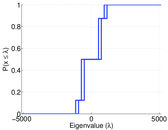### Spectral distribution of the normalized adjacency matrix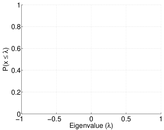### Spectral distribution of the Laplacian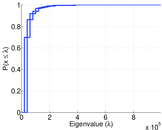### Spectral graph drawing based on the adjacency matrix### Spectral graph drawing based on the Laplacian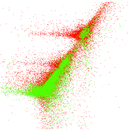### Spectral graph drawing based on the normalized adjacency matrix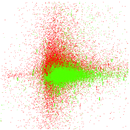### Degree assortativity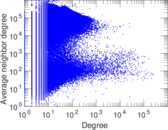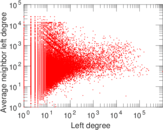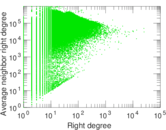### Hop distribution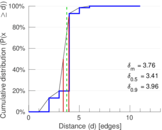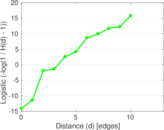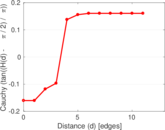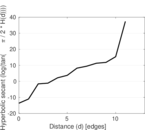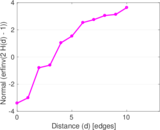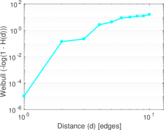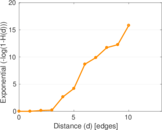### Edge weight/multiplicity distribution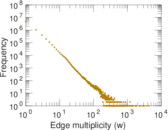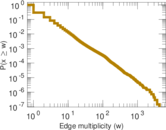### Temporal distribution